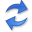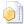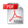Causal Inference on Multivariate Mixed-Type Data

Abstract. How can we discover whether $$X$$ causes $$Y$$, or vice versa, that $$Y$$ causes $$X$$, when we are only given a sample over their joint distribution? How can we do this such that $$X$$ and $$Y$$ can be univariate, multivariate, or of different cardinalities? And, how can we do so regardless of whether $$X$$ and $$Y$$ are of the same, or of different data type, be it discrete, numeric, or mixed? These are exactly the questions we answer in this paper.

We take an information theoretic approach, based on the Minimum Description Length principle, from which it follows that first describing the data over extit{cause} and then that of effect given cause is shorter than the reverse direction. Simply put, if $$Y$$ can be explained more succinctly by a set of classification or regression trees conditioned on $$X$$, than in the opposite direction, we conclude that $$X$$ causes $$Y$$. Empirical evaluation on a wide range of data shows that our method, Crack, infers the correct causal direction reliably and with high accuracy on a wide range of settings, outperforming the state of the art by a wide margin.

## Implementationthe C++ source code (January 2019) by Alexander Marx.

## Related PublicationsMarx, A & Vreeken, J Causal Inference on Multivariate and Mixed Type Data. In: Proceedings of the European Conference on Machine Learning and Principles and Practice of Knowledge Discovery in Data (ECMLPKDD), Springer, 2018. (25% acceptance rate)Marx, A & Vreeken, J Causal Inference on Multivariate Mixed Type Data. Technical Report 1702.06385, arXiv, 2017.